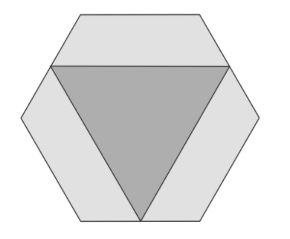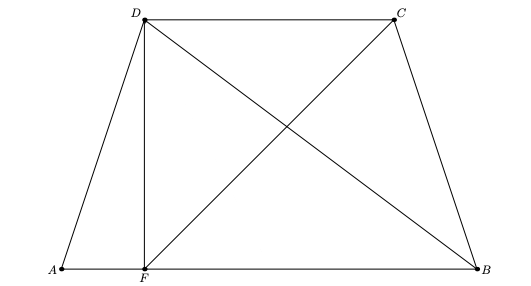# MT #6: Full-syllabus

## Part A: Short-answer type questions

Submission file: Write answers to all the ten questions on a single sheet of paper. Email a picture of your answer sheet. Name the file as PartA.jpg.

For this part, answers must be written without any explanation.

1. Find a non-constant polynomial that divides all the polynomials in the set: $\mathcal{P} = \{ P_k: P_k(x) = x^{3k+2}+x+1, k\in \mathbb{N} \}$
2. Let $$\alpha_1,\ldots,\alpha_n$$ denote the $$n$$ roots of unity. Let $$\alpha_1=1$$. What is the value of the following product? $\prod_{i=2}^n (1-\alpha_i)$
3. We have a regular hexagon whose area is 1 sq.unit. A triangle is formed by joining the midpoints of alternate sides as shown below. What is the area of the triangle?4. Let $$\displaystyle f(x) = \frac{x^2e^{x^2}}{1-x^2}$$. Find the $$6$$th derivative of $$f$$ evaluated at 0, that is, $$f^6(0)$$.
5. The number of real valued solutions to the equation $$e^x=\sin x$$ is:
1. 0
2. 1
3. 2
4. 4
5. none of the above.
6. Find a line $$l$$ that intersects the four lines given below. The lines are in $$\mathbb{R}^3$$. Express the equation of $$l$$ in the parameterized form as given for $$l_i$$s.
\begin{align*} l_1: &\; (1,0,0) + t(0,0,1) \\ l_2: &\; (0,1,0) + t(1,0,0) \\ l_3: &\; (0,0,1) + t(0,1,0) \\ l_4: &\; (0,0,0) + t(6,6,-1) \end{align*}
7. Suppose $$a_i$$ and $$b_i$$ are real numbers such that $$\sum_1^\infty a_i^2$$ and $$\sum_1^\infty b_i^2$$ converge. Which of these statements is true?
1. The sequence $$\sum_1^\infty |a_i-b_i|$$ converges.
2. The sequence $$\sum_1^\infty |a_i-b_i|^{3/2}$$ converges.
3. The sequence $$\sum_1^\infty (a_i-b_i)^2$$ converges.
4. The sequence $$\sum_1^\infty (a_i-b_i)^3$$ converges.
8. Let $$\phi(n)$$ denote the number of positive integers less than $$n$$ that are relatively prime to $$n$$. In other words, $$\phi(n)$$ counts all $$m$$ such that $$\operatorname{gcd}(m, n)=1$$.

The number $$n=220358$$ is a product of three primes $$p$$, $$q$$ and $$r$$. We also know that $$\phi(n) = 109480.$$

Find the values of $$p$$, $$q$$ and $$r$$.

9. Calculate the determinant of the matrix given below: \begin{bmatrix} 1 & 2 & 3 & 4 & 5 & 6\\ 2 & 2 & 3 & 4 & 5 & 6\\ 3 & 3 & 3 & 4 & 5 & 6\\ 4 & 4 & 4 & 4 & 5 & 6\\ 5 & 5 & 5 & 5 & 5 & 6\\ 6 & 6 & 6 & 6 & 6 & 6\\ \end{bmatrix}

10. Suppose $$S=\{1,2,3,4,5,6,7\}$$. Find the number of pairs $$(A,B)$$ that can be formed such that $$A \subseteq S$$ and $$B\subset A$$.

## Part B: Subjective questions

Submission files: Each question in this part must be answered on a page of its own. Name the files as B1.jpg, B2.jpg, etc. In case you have multiple files for the same question, say B4, name the corresponding files as B4-1.jpg, B4-2.jpg, etc.

Clearly explain your entire reasoning. No credit will be given without reasoning. Partial solutions may get partial credit.

B1. Let $$p(x)=a_{0}+a_{1} x+a_{2} x^{2}+a_{3} x^{3}$$ be a polynomial with integer coefficients such that $$a_{0}$$ and $$a_{3}$$ are odd numbers.

1. Suppose $$p$$ has a rational root $$\frac{p}{q}$$, where $$\text{gcd}(p,q)=1$$. Prove that both $$p$$ and $$q$$ must be odd.
2. Suppose $$a_0,a_3$$ and $$a_0+a_1+a_2+a_3$$ are all odd. Prove that $$f$$ has no rational roots.

B2. By induction or otherwise, show that: $\binom{n}{2} ! >\; 2^{n^2}$ for all $$n\geq 6$$.

B3. We are given two positive integers $$a>2$$ and $$n$$ such that the following $$n+1$$ numbers are all composite numbers: $a,a+1,a+2,\ldots,a+n$ Prove that there exists an integer $$t>1$$ such that the following consecutive numbers are all composite: $a^t,a^t+1,a^t+2,\ldots,a^t+n$

B4. Consider the trapezoid $$ABCD$$ in which $$|AD|=|BC|$$. Prove that the centroid of the triangle $$ABD$$ lies on the line $$CF$$. Here, point $$F$$ is the projection of $$D$$ on $$AB$$.B5. Let $$\pi$$ denote a permutation of numbers from $$1$$ to $$12$$. We denote the number at the $$i$$th position by $$\pi(i)$$. For example, if the permutation is $$[1,12,11,2,3,4,5,6,7,8,9,10]$$, then $$\pi(1) = 1$$, $$\pi(2)=12$$, $$\pi(3)=11$$, etc. Count the number of permutations $$\pi$$ that satisfy the following condition: $\pi(i) < \pi(i+1) \;\;\text{for } i \in \{1,2,4,5,7,8,9,11\}$ Write your answer as a single number (without any factorials or binomials).

B6. We have a function $$f:\mathbb{R}\rightarrow\mathbb{R}$$ that is twice differentiable and satisfies the following equation: $\int_{x-y}^{x+y} f(t) dt = 2yf(x)$ Prove that $$f(x)=ax+b$$ for some $$a,b\in\mathbb{R}$$.